# AP 9th Physical Science Mock Test 2023 for Chapter-3 “Laws Of Motion” Online Practice Test

1. Internal forces
2. External forces
3. Momentum gives a measure of
4. Product of mass and acceleration measure
5. SI unit of force is
6. N/kg is a unit of
7. The rate of change of momentum w.r.t time is measured in
8. Kg m/s2 is the SI unit of
9. A gun recoils after firing. The reason explained by
10. A jet engine or a rocket works on the principle of
11. A ball of mass 'm' moves perpendicularly to a wall with a speed 'v' strikes it and rebounds with the same speed , then the change in momentum is
12. The force that always opposes motion of objects
13. An iron-ball moving with a in initial velocity 'u' changes its velocity to 'v' in time t sec , if the mass of a ball  is 'm' kg the force acting on it F =
14. Mass in motion as described by Newton is
15. In an isolated system the total ................. is conserved
16. A non zero net force acting on a body disturbs the state of
17. ....... is a property of matter that resist changes in its state of rest of motion
18. The product of net force and interaction time is called ................. of net force
19. Identify the correct statement regarding Aristotle's belief
20. Rate of change of momentum is proportional to
21. The product of mass and velocity is called
22. When we drop an egg on the concrete floor it will break because
23. A: Action reaction pair in Newton's third law always represents forces acting on two bodies simultaneously
B: Newton's first and second laws of motion apply to a single body
24. Assertion (A) : Pace bowler Bumrah runs in from a long distance before he bowls
Reason (R) : To acquire dynamic inertia
25. An unbalanced force causes a change in................ of an object
26. As the boatman jumps in forward directions , the boat moves
27. A passenger in a moving train tosses a coin which falls infront of him means that the motion is
28. take a block of ice and place it on a smooth surface , push the block with some net force . Now increase net force and push it again . the outcome of the experiment is
29. Make a stack of carrom cons on the carrom board . Give a sharp hit at the bottom of the stack with the striker , the law which represent the above experiment is
30. One of the following is not a force
31. If Fnet = 0 , then velocity of the object is
32. The measurement of inertia of an object is
33. Physical quantity for force
34. Physical quantity for momentum
35. Physical quantity of impulse is given by
36. SI unit of momentum
37. SI unit for force
38.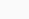=
39. One newton is equal to the
40. Inertia is a property of matter that resists changes in its state of motion or rest . it depends on the mass of the object
From the above statement , arrange the following animals in increasing order of inertia
a. elephant , b. lion , c. dog , d.rat
41. A rocket moves in an empty space at a speed of 6 km/s . the speed of bolt separated from the rocket is
42. A suitcase kept in a moving bus moves forward when the bus
43. Safe way to catch the fast moving cricket ball is , while catching
44. A passenger in a moving train tosses a coin which falls behind him , it means the motion of the train is
45. When 2.5 kg of hero's hand hits the villain of 80 kg who is at rest , the villain moves with 12.5 ms-1 and hits the transformer near by . What will be the speed of hero's hand compared to the bullet fired from the gun ?
46. All the bodies have the same inertia
47. ..................... is the force which opposes the relative motion between two objects which are in contact
48. A and B are two bodies .A exerts a force on B by F due north , what is the force on A by B ?
49. The symbol for linear momentum is
50. 'J' is symbol for
51. Choose the correct matching for the following
1. SI unit of momentum          ( )   a)1 kg/ms-2
2. SI unit of force                      ( )   b)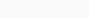3.=.....                                   ( )   c)kg ms-1
4. One Newton                          ( )   d)Newton
52. Match the physical quantities given in set - A with proper units in Set - B.

Set - A                                                  Set - B
1. Force                                     ( )            x) kg ms2
2. Momentum                          ( )            y) kg ms-2
3. Impulse                                 ( )            z) kg ms-1
53. Which of the following represents correct diagram of atwood machine ?
54. The following shows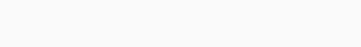55. What does the following indicates ?56. The materials required for following experiment is57. Newtons first law explains about
58. p = mv , here m means
59. The body which is in motion always tries to move in same direction until some force act on it , this property is known as
60. Sate the following61. A : A truck moves with a certain speed and collides a wall and comes to rest
B : A truck moves with a certain speed and collides a heap of grass and comes to rest
In which situation lorry damaged more?
62. Symbol for newton
63. Match the following
1. Newton       ( ) a.P
2. Mass            ( ) b.N
3. Momentum( ) c.M
64. ................. is the string always acts away from the body to which it is attached
65. A: Fnet =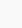B: Fnet = ma
66. A system is said to be isolated system when
67. What does the following ?68. Newton proposed 3 fundamentals laws which explain the connection between force and change in motion .These 3 laws are known as
69. Inertia depends on ....... object
70. In which property of matter that resists changes in its state of motion or rest ?
71. Linear momentum of body is product of the
72. Linear momentum of body is product of the
73. SI unit for P is
74. Momentum has CGS unit is
75. In the absence of net external force on the system , the momentum of the system remains unchanged this is called
76. The impulse of force is equal to the change in ............................... that an object experiences during an interaction
77. Impulse is a
78. CGS unit for impulse
79. Forces exerted over a limited time are called
80. ........... is the tendency of a body to continue in its state of rest
81. ........... is the tendency of a body to continue in its state of uniform motion in a straight line
82. The inability of a body to continue in its state of uniform motion in straight line is
83. Two people push the car for 3s with a combined net force of 200 N , then the impulse provided for car is
84. What is the momentum of a 6.0 kg bowling ball with a velocity of 2.2 m/s ?
85. The objects have masses 8 kg , 25 kg and 50 kg respectively then which one has more inertia ?
86. When a carpet is beaten with a stick , dust comes out of it due to
87. A passenger in moving train tosses a coin which falls behind him.It means that the motion of the train is
88. What force is required to produce an acceleration of 3m/s2 in an object of mass 0.7 kg ?
89. CGS unit for force is
90. A : Mass is scalar quantity
B : Force is vector quantity
91. Choose the true option
1. Mass                          ( ) a. Newton-second
2. Linear momentum ( ) b. Kilogram
3. Force                         ( ) c. N-s
4. Impulse                    ( ) d. Newton
92. Match the correct symbol
1. Impulse                   ( ) a. F
2. Force                        ( ) b. J
3. Mass                         ( ) c. p
4. Linear momentum( ) d. m
93. What is the velocity of a small object that has separated from the rocket moving in free space with velocity 10 km/s ?
94. The force exerted by the earth on the ball is 8N . What is the force on the earth by he ball ?
95. The following is example for96. It is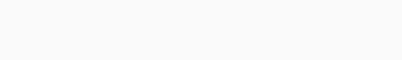97. The following is used to show the98. Which of the following are not example for Newton's first law of motion ?
99. Which of the following are not Newton's second law of motion ?